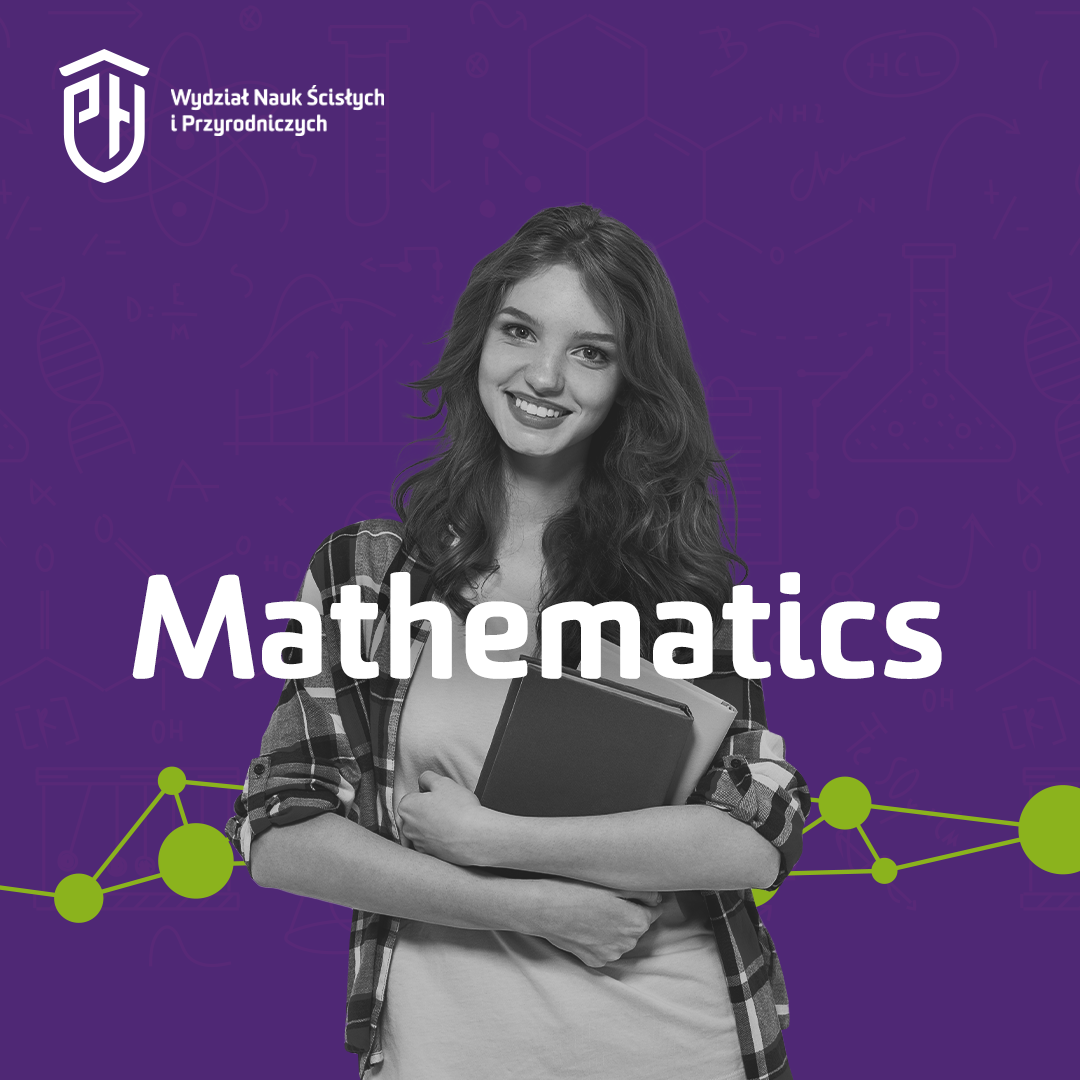## Kandydaci2ND LEVEL  DEGREE IN  MATHEMATICS.  The 2-year period of studies includes: calculus, complex and functional analysis, logic, algebra and number theory, topology, differential equations, stochastic processes, and computational mathematics, i.e. packages Mathematica and Statistica. Students are prepared to continue their studies to obtain doctorate degree.

A graduate of applied mathematics acquires the basic knowledge in theoretical and applied mathematics. He is enabled to do mathematical proofs, present and formulate mathematical problems, investigate mathematical models necessary in the field of applications, apply IT knowledge. The graduates are prepared to work in commercial and financial companies, computing centers and statistical offices.

A graduate of financial mathematics acquires the basic knowledge in theoretical and applied mathematics in economy and finance. He is enabled to do mathematical proofs, present and formulate mathematical problems, investigate mathematical models and estimate, predict and simulate economical processes. He is prepared to financial management and apply  IT knowledge. The graduates can work in financial companies, banks, stock markets, insurance and commercial industry.

The University reserves the right not to open a department or specialty when the number of accepted candidates is too low.

Wydział Nauk Ścisłych i Przyrodniczych
Instytut Matematyki
ul. 3 Maja 54
08-110 Siedlce